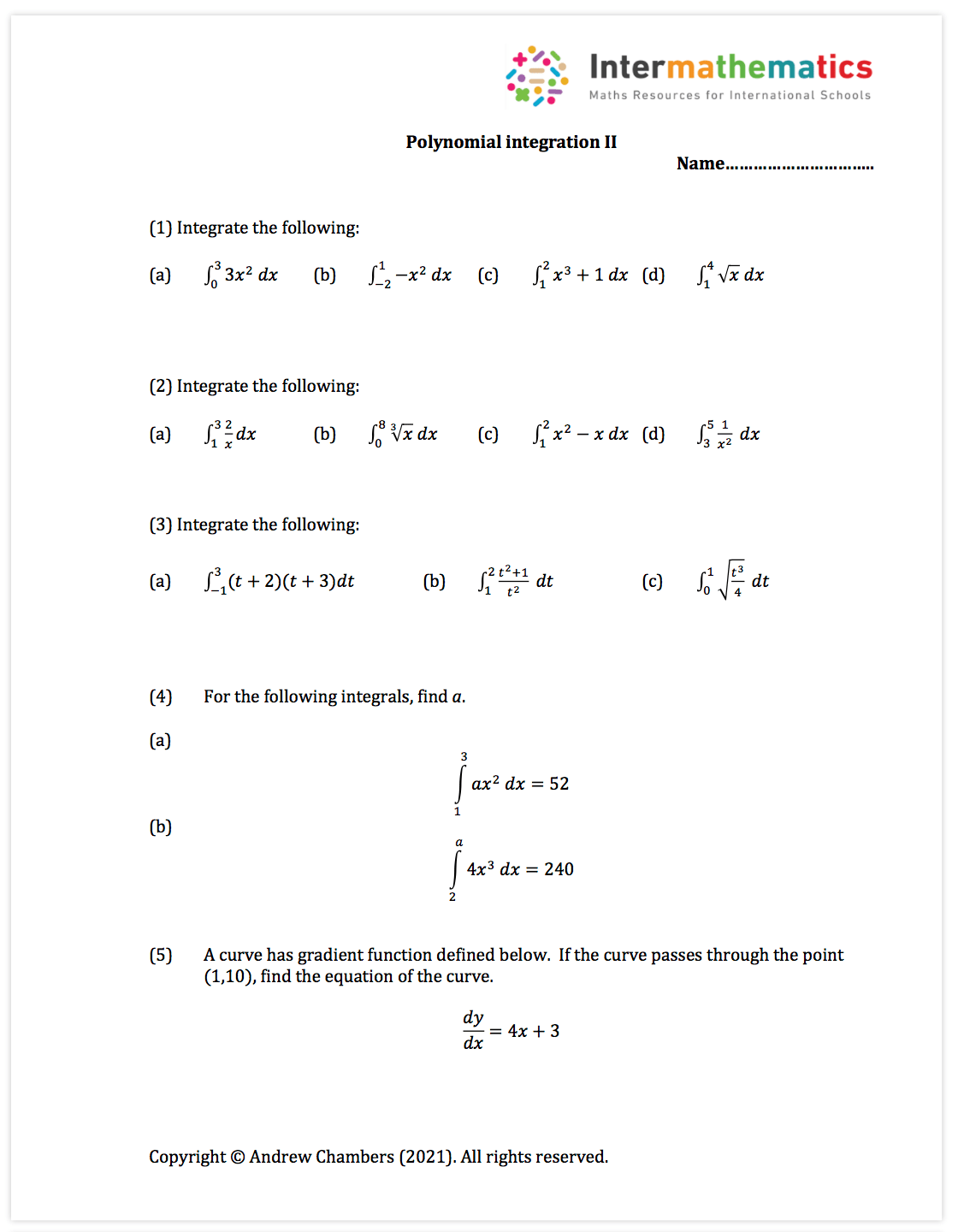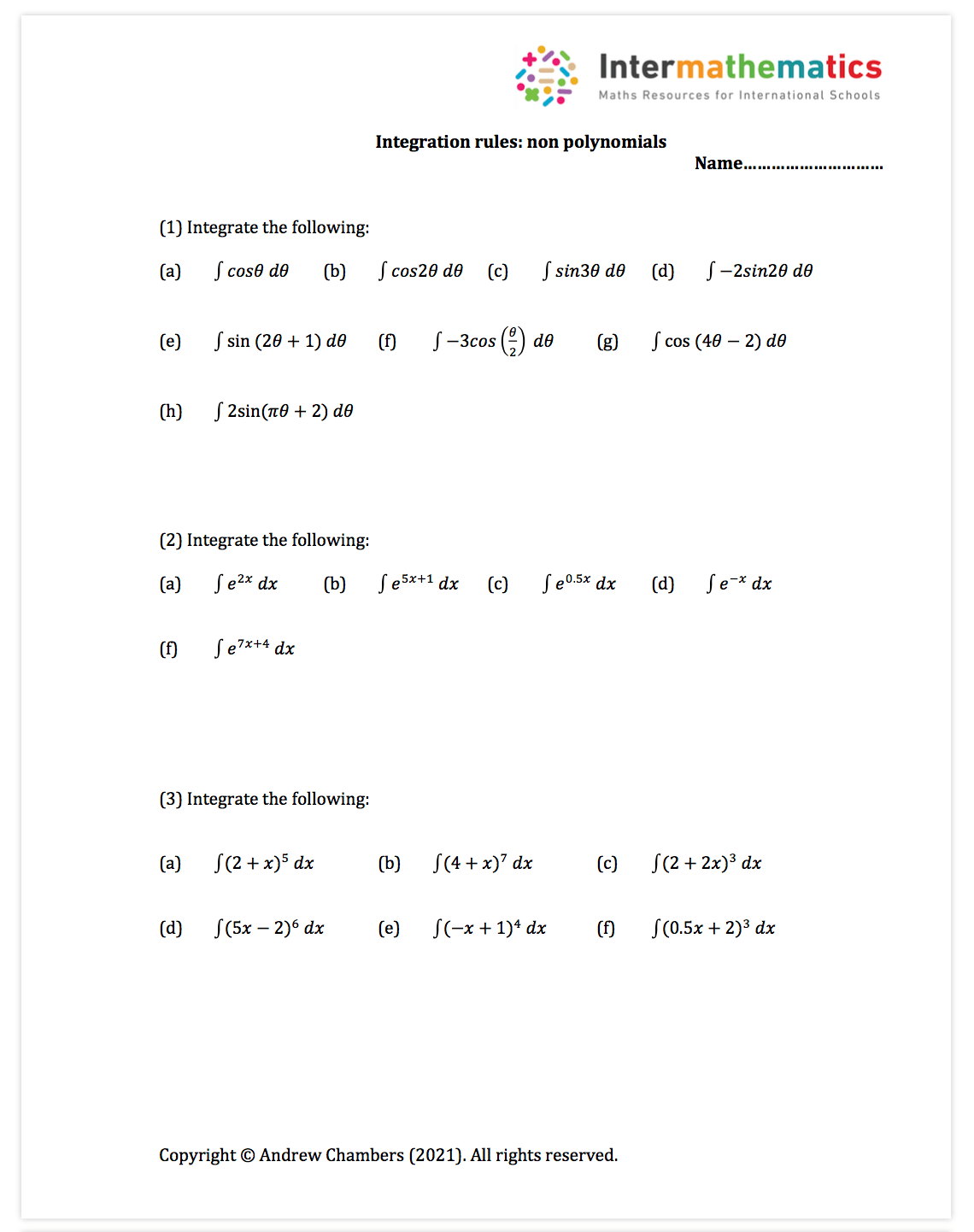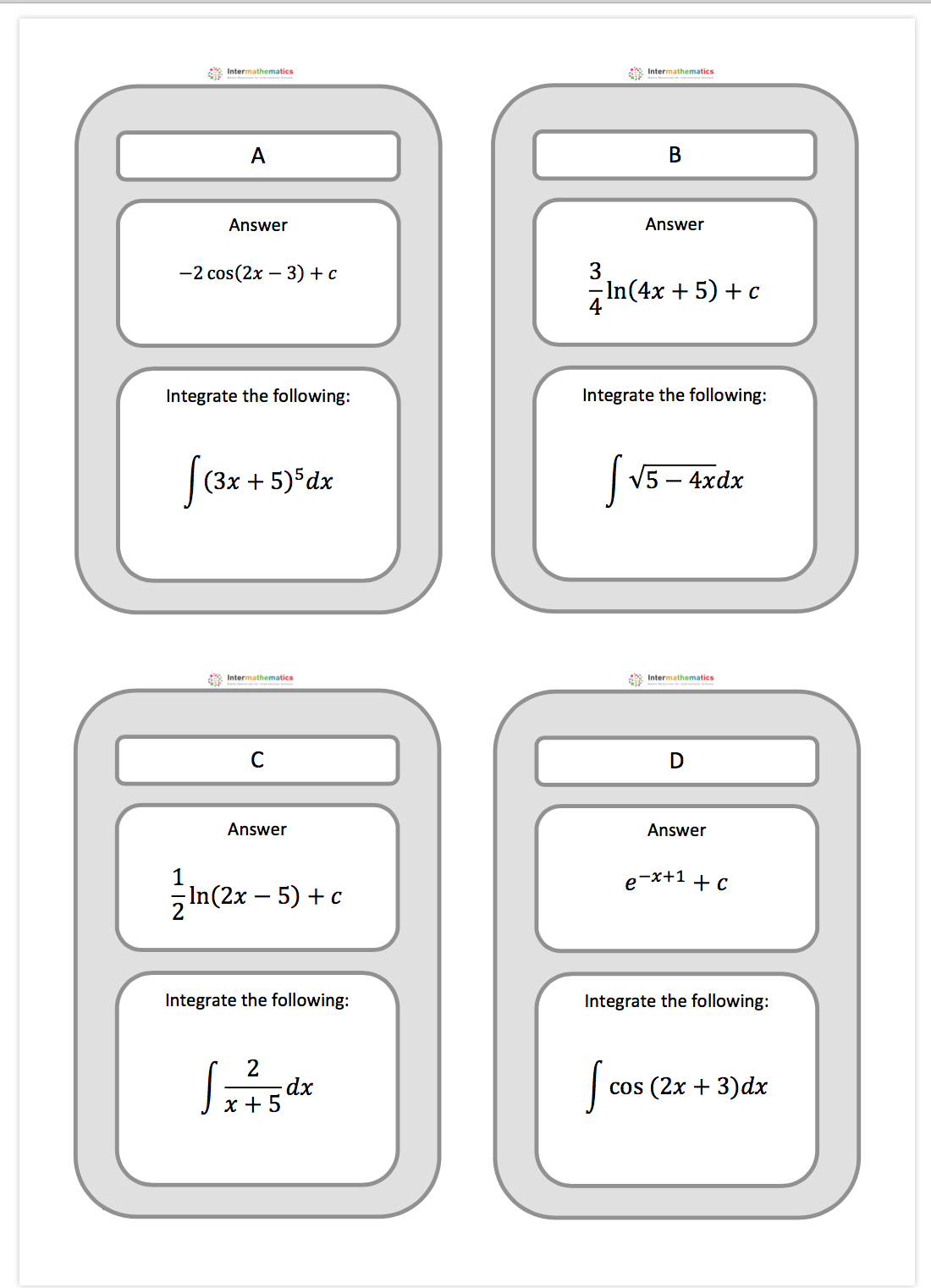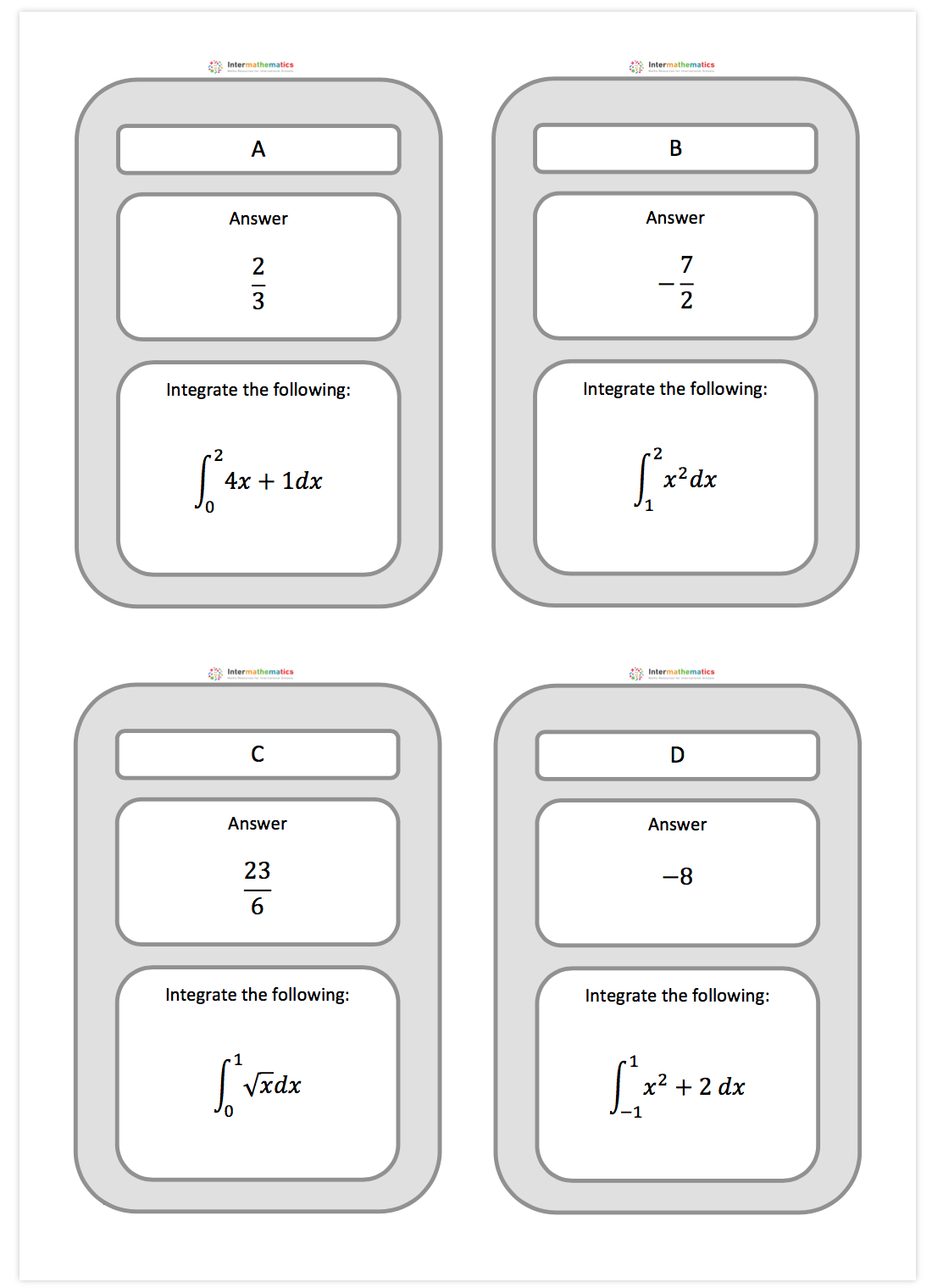# Integration Fundamentals

Integration Fundamentals

An introduction to the basic ideas on integration

Syllabus content: SL 5.5, SL 5.10, SL 5.11

I have made 6 worksheets to help with these topics.  They are:

1. Polynomial integration
2. Polynomial integration 2
3. Area between 2 curves and FTC
4. Integration rules for non polynomials
5. Treasure hunt: Integration non polynomials
6. Treasure hunt: Integration polynomials

You can scroll down below to see the pictures of the questions.  You can download both the questions and mark scheme from the bottom of the page.

1. Polynomial integrationNote:  This is a short worksheet which can be used in lesson or for homework and gives some practice in basic polynomial integration.  Syllabus point: SL 5.5

2. Polynomial integration part 2Note:  This is a short worksheet which can be used in lesson or for homework and gives some more practice in basic polynomial integration.  Syllabus point: SL 5.5

3. Area between 2 curves and FTCNote:  This is a short worksheet which can be used in lesson or for homework and gives some practice in finding area between curves.  Syllabus point: SL 5.5 and SL 5.11

4. Integration rules for non polynomialsNote:  This is a short worksheet which can be used in lesson or for homework and gives some practice in basic non-polynomial integration.  Syllabus point: SL 5.10.

5. Treasure hunt: Integration non polynomialsNote:  This is an activity which can be done in pairs or as a whole class activity.  Students integrate using guess and check methods. Syllabus point:  5.10.

6. Treasure hunt: Integration polynomialsNote:  This is an activity which can be done in pairs or as a whole class activity.  Students find definite integrals of polynomials.  Syllabus point:  5.5.